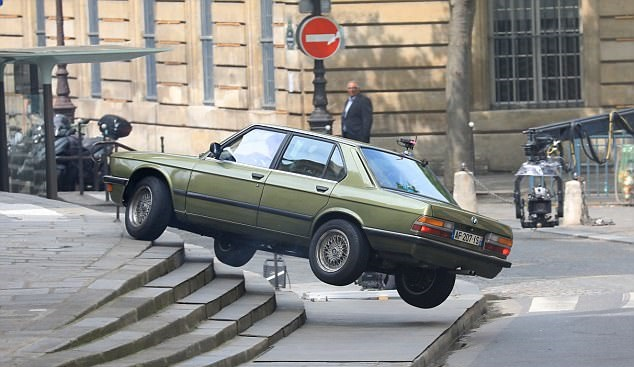## Problem 4: Car Convergence ChaosA nuclear bomb has been set up somewhere in Vancouver! Ethan Hunt and Solomon Lane have just learned of its location, and are each desperate to reach it first. If Ethan arrives on the scene first, he'll be able to shut it down, while if Solomon beats him to it, he'll surely detonate it to take the entire city down with him!

Vancouver has N (1 ≤ N ≤ 5000) traffic intersections lined up in a row, numbered from 1 to N in order. Ethan is currently at some intersection E, while Solomon is at a different intersection S, such that E < S and SE is even. The bomb happens to be located at the intersection M which is equidistant from both E and S (such that M = (E + S)/2).

Ethan will drive a car through the sequence of intersections EE + 1 → … → M − 1 → M, while Solomon will drive through the sequence SS − 1 → … → M + 1 → M, until at least one of them arrives at intersection M. Though they're both in quite the hurry, disobeying traffic laws would be too reckless, so they'll patiently wait at each intersection until the lights turn green. When a car is at an intersection i, it must wait there for Ti (1 ≤ Ti ≤ 100,000) minutes before driving onwards to an adjacent intersection (either intersection i − 1 or i + 1), at which point the car will instantly move to that intersection.

For example, if E = 3 and S = 7, Ethan will spend the first T3 minutes at intersection 3 and the next T4 minutes at intersection 4, before reaching the bomb (intersection 5) after a total of T3 + T4 minutes. Meanwhile, Solomon will spend T7 minutes at intersection 7 followed by T6 minutes at intersection 6, and reach the bomb after T7 + T6 minutes. If T3 + T4 = T7 + T6, then both cars will reach the bomb. Otherwise, the slower car will stop driving as soon as the faster car reaches the bomb.

Meanwhile, Alan Hunley, the secretary of the IMF, will be monitoring the action closely. He'd like to keep track of how many times the identity of the agent currently closer to the bomb changes. To do so, he'll first form a Leader List, which has one entry for each minute (including both the very beginning, and the moment when a car reaches the bomb) indicating who is closer to the bomb (distance-wise) at that point in time. The distance between a car at some intersection i and the bomb (at intersection M) is |Mi|. Each entry in the Leader List is either "`Ethan`", "`Solomon`", or "`Neither`" (if they're both equidistant from the bomb), and the first entry (at minute 0) is always "Neither". Alan will then remove all "`Neither`" entries from the Leader List (which may cause it to become empty). Finally, he will count the number of entries in the resulting Leader List which are either different than their preceding entry, or have no preceding entry. This count is formally known as the CCCC (Closer Car Change Count).

For example, if the initial Leader List is ["`Neither`", "`Solomon`", "`Ethan`", "`Neither`", "`Ethan`", "`Ethan`", `Solomon`"], then Alan will reduce this to ["`Solomon`", "`Ethan`", "`Ethan`", "`Ethan`", "`Solomon`"], and determine its CCCC to be 3 (due to its 1st, 2nd, and 5th entries).

Due to some complex quantum time singularity science, the scenario described above will actually play out separately in one or more parallel universes, just with the initial state varying. Specifically, there is one parallel universe for each possible valid pair of starting intersections E and S (such that E < S and SE is even). Considering each possible (E, S) pair independently, and computing its resulting CCCC value, what's the sum of all of these CCCC values?

In test cases worth 8/42 of the points, N ≤ 300 and Ti ≤ 20 for each i.
In test cases worth 18/42 of the points, Ti ≤ 20 for each i.

### Input Format

The first line of input consists of a single integer, N.
The next line consists of N integers, T1..N.

### Output Format

Output a single integer, the sum of all parallel universes' CCCC values.

```4
5 6 5 3
```

```1
```

```7
7 3 6 2 5 2 8
```

```9
```

### Sample Explanation

In the first case, there are two possible (E, S) pairs: (1, 3) and (2, 4). The CCCC value for the first pair is 0, as the both Ethan and Solomon will be 1 intersection away from the bomb for minutes 0..4, and then 0 intersections away at minute 5, resulting in an empty Leader List. The CCCC value for the second pair is 1, as Solomon will become 0 intersections away from the bomb at minute 3 while Ethan is still 1 intersection away from it, resulting in a Leader List of ["`Solomon`"]. The sum of these CCCCs is then 0 + 1 = 1.

In the second case, there are 9 possible (E, S) pairs, with the following CCCC values:

• (1, 3): 1
• (1, 5): 1
• (1, 7): 2
• (2, 4): 1
• (2, 6): 1
• (3, 5): 1
• (3, 7): 1
• (4, 6): 0
• (5, 7): 1

The sum of these CCCCs is then 1 + 1 + 2 + 1 + 1 + 1 + 1 + 0 + 1 = 9.

Point Value: 25 (partial)
Time Limit: 4.00s
Memory Limit: 256M